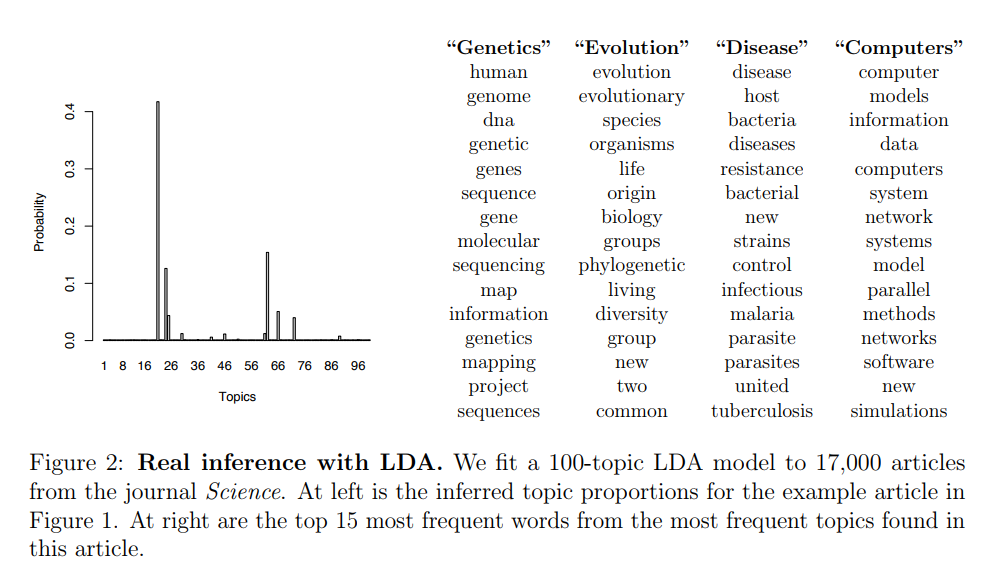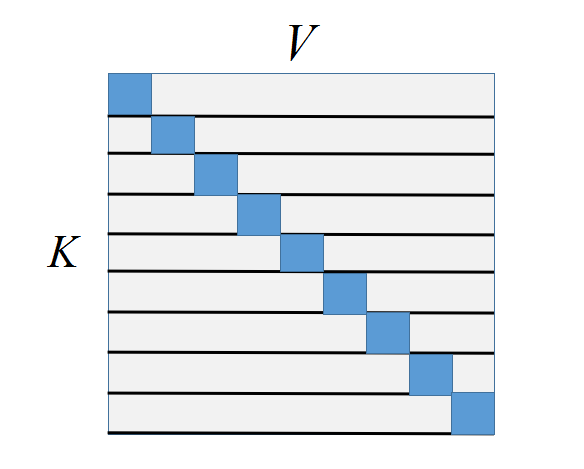# LDA(Latent Dirichlet Allocation)主题模型

10 篇文章 3 订阅

LDA于2003年由 David Blei, Andrew Ng和 Michael I. Jordan提出，因为模型的简单和有效，掀起了主题模型研究的波浪。虽然说LDA模型简单，但是它的数学推导却不是那么平易近人，一般初学者会深陷数学细节推导中不能自拔。于是牛人们看不下去了，纷纷站出来发表了各种教程。国内方面rickjin有著名的《LDA数学八卦》，国外的Gregor Heinrich有著名的《Parameter estimation for text analysis》。其实有了这两篇互补的通俗教程，大家沉住心看个4、5遍，基本就可以明白LDA为什么是简单的了。那么其实也没我什么事了，然而心中总有一种被大牛点播的豁然开朗的快感，实在是不吐不快啊。

# 什么是主题

$\left\{科比，篮球，足球，奥巴马，希拉里，克林顿\right\}$

{} { 体 育 ， 政 治 }
LDA说体育这个主题就是：
{:0.3:0.3:0.3:0.03:0.03:0.04} { 科 比 : 0.3 ， 篮 球 : 0.3 ， 足 球 : 0.3 ， 奥 巴 马 : 0.03 ， 希 拉 里 : 0.03 ， 克 林 顿 : 0.04 }
(数字代表某个词的出现概率)，而政治这个主题就是：
{:0.03:0.03:0.04:0.3:0.3:0.3} { 科 比 : 0.03 ， 篮 球 : 0.03 ， 足 球 : 0.04 ， 奥 巴 马 : 0.3 ， 希 拉 里 : 0.3 ， 克 林 顿 : 0.3 }
LDA就是这样说明什么是主题的，竟说得我无言以对，细思之下也是非常合理。

# 文章在讲什么

《 体 育 快 讯 》 ， 《 娱 乐 周 报 》

:[,,,,,....,,] 《 体 育 快 讯 》 是 这 样 的 : [ 废 话 , 体 育 , 体 育 , 体 育 , 体 育 , . . . . , 娱 乐 , 娱 乐 ]
:[,,,,,....,,] 《 娱 乐 周 报 》 是 这 样 的 : [ 废 话 , 废 话 , 娱 乐 , 娱 乐 , 娱 乐 , . . . . , 娱 乐 , 体 育 ]

# 文章是如何生成的

= 灵 感 = 随 机

1. 确定主题和词汇的分布
2. 确定文章和主题的分布
3. 随机确定该文章的词汇个数 N N $N$
4. 如果当前生成的词汇个数小于$N$$N$执行第5步，否则执行第6步
5. 由文档和主题分布随机生成一个主题，通过该主题由主题和词汇分布随机生成一个词，继续执行第4步
6. 文章生成结束

# LDA数学分析

## 多项式分布

${C}_{n}^{k}{p}^{k}\left(1-p{\right)}^{n-k}$

n!n1!n2!,....,nk!p1n1p2n2....pknk n ! n 1 ! n 2 ! , . . . . , n k ! p 1 n 1 p 2 n 2 . . . . p k n k
LDA出来说话了： 主题和词汇的分布就是多项式分布！因为一个主题里面不同词汇出现的概率不同，如果只有1个词汇，那么它就是二项分布，但是词汇不可能只有1个，所以它理所当然就是符合多项式分布。这是挺合理的假设，反正我们现在研究的内容是为词汇按主题给出不同的概率分布，其实也就是给出不同词汇在不同主题下的出现比例，我们并不关系词汇之间的顺序关系，所以多项式分布已经很好地刻画出这种关系了。LDA又说： 文章和主题之间的分布也是符合多项式分布！因为一篇文章要确定不同主题的出现概率，和主题要确定每个词汇的出现概率是完全可以类比的！思考一下我们也接受了LDA的说法，接下来我们介绍一些记号：

• V V $V$代表我们字典的词汇个数
• $K$$K$代表主题的个数
• M M $M$代表文章的个数
• $\stackrel{\to }{{\varphi }_{k}}$$\vec{\phi_k}$代表第k个主题的多项式分布参数，长度为 V V $V$，因此$\mathrm{\Phi }$$\Phi$是一个 KV K ∗ V $K*V$的矩阵，每一行代表一个主题的多项式分布参数
• θm θ m → $\vec{\theta_m}$代表第 m m $m$篇文章的多项式分布参数，长度为$K$$K$，因此 Θ Θ $\Theta$是一个 MK M ∗ K $M*K$的矩阵，没一行代表一篇文章的多项式分布参数
• Nm N m $N_m$代表第 m m $m$篇文章的长度
• ${z}_{m,n}$$z_{m,n}$代表第 m m $m$篇文章第$n$$n$个词由哪个主题产生的
• wm,n w m , n $w_{m,n}$代表第 m m $m$篇文章第$n$$n$个词

zMulti(z|θm) z ∼ M u l t i ( z | θ m → )

wMulti(w|ϕk) w ∼ M u l t i ( w | ϕ k → )

## Dirichlet分布

ϕ⃗ Dirichlet(α⃗ ) ϕ → ∼ D i r i c h l e t ( α → )
θ⃗ Dirichlet(β⃗ ) θ → ∼ D i r i c h l e t ( β → )

$p\left(\stackrel{\to }{{z}_{m}},\stackrel{\to }{{w}_{m}},\stackrel{\to }{{\theta }_{m}},\mathrm{\Phi }|\stackrel{\to }{\alpha },\stackrel{\to }{\beta }\right)=\prod _{n}^{{N}_{m}}p\left({w}_{m,n}|\stackrel{\to }{{\varphi }_{{z}_{m,n}}}\right)p\left({z}_{m,n}|\stackrel{\to }{{\theta }_{m}}\right)p\left(\stackrel{\to }{{\theta }_{m}}|\stackrel{\to }{\alpha }\right)p\left(\mathrm{\Phi }|\stackrel{\to }{\beta }\right)$

$p\left(\stackrel{\to }{{w}_{m}}|\stackrel{\to }{\alpha },\stackrel{\to }{\beta }\right)\phantom{\rule{0ex}{0ex}}={\int }_{{\theta }_{m}}{\int }_{\mathrm{\Phi }}{\int }_{\stackrel{\to }{{z}_{m}}}\prod _{n}^{{N}_{m}}p\left({w}_{m,n}|\stackrel{\to }{{\varphi }_{{z}_{m,n}}}\right)p\left({z}_{m,n}|\stackrel{\to }{{\theta }_{m}}\right)p\left(\stackrel{\to }{{\theta }_{m}}|\stackrel{\to }{\alpha }\right)p\left(\mathrm{\Phi }|\stackrel{\to }{\beta }\right)\phantom{\rule{0ex}{0ex}}={\int }_{{\theta }_{m}}{\int }_{\mathrm{\Phi }}\sum _{\stackrel{\to }{{z}_{m}}}\prod _{n}^{{N}_{m}}p\left({w}_{m,n}|\stackrel{\to }{{\varphi }_{{z}_{m,n}}}\right)p\left({z}_{m,n}|\stackrel{\to }{{\theta }_{m}}\right)p\left(\stackrel{\to }{{\theta }_{m}}|\stackrel{\to }{\alpha }\right)p\left(\mathrm{\Phi }|\stackrel{\to }{\beta }\right)\phantom{\rule{0ex}{0ex}}={\int }_{{\theta }_{m}}{\int }_{\mathrm{\Phi }}\sum _{{z}_{m1}}\sum _{{z}_{m2}}...\sum _{{z}_{m{N}_{m}}}\prod _{n}^{{N}_{m}}p\left({w}_{m,n}|\stackrel{\to }{{\varphi }_{{z}_{m,n}}}\right)p\left({z}_{m,n}|\stackrel{\to }{{\theta }_{m}}\right)p\left(\stackrel{\to }{{\theta }_{m}}|\stackrel{\to }{\alpha }\right)p\left(\mathrm{\Phi }|\stackrel{\to }{\beta }\right)\phantom{\rule{0ex}{0ex}}={\int }_{{\theta }_{m}}{\int }_{\mathrm{\Phi }}\prod _{n}^{{N}_{m}}\sum _{{z}_{mn}}p\left({w}_{m,n}|\stackrel{\to }{{\varphi }_{{z}_{m,n}}}\right)p\left({z}_{m,n}|\stackrel{\to }{{\theta }_{m}}\right)p\left(\stackrel{\to }{{\theta }_{m}}|\stackrel{\to }{\alpha }\right)p\left(\mathrm{\Phi }|\stackrel{\to }{\beta }\right)$

## Gibbs 采样算法

### 采样算法的思想

x⃗ =[x1,x2,x3,...,xi,...,xn] x → = [ x 1 , x 2 , x 3 , . . . , x i , . . . , x n ]

x⃗ (1)=[0,0,0,0,....0]x⃗ (2)=[1,1,0,0,....0]x⃗ (3)=[1,0,0,0,....0]x⃗ (4)=[1,1,0,0,....0].......x⃗ (N)=[1,1,0,0,....0] x → ( 1 ) = [ 0 , 0 , 0 , 0 , . . . .0 ] x → ( 2 ) = [ 1 , 1 , 0 , 0 , . . . .0 ] x → ( 3 ) = [ 1 , 0 , 0 , 0 , . . . .0 ] x → ( 4 ) = [ 1 , 1 , 0 , 0 , . . . .0 ] . . . . . . . x → ( N ) = [ 1 , 1 , 0 , 0 , . . . .0 ]

$p\left({x}_{1}=1,{x}_{2}=1,{x}_{3}=0,....,{x}_{n}=0\right)=\frac{K}{N}$

### 神奇的Gibbs采样

p(x⃗ ) p ( x → )

p(xi|x¬i) p ( x i | x ¬ i → )

• 随机初始化 x⃗ 0={x01,x02,...,x0N} x → 0 = { x 1 0 , x 2 0 , . . . , x N 0 } $\vec{x}^0=\{x_1^0,x_2^0,...,x_N^0\}$
• 对于 t=1,2,3,4,….T:
• xt1p(x1|xt1¬1) x 1 t ∼ p ( x 1 | x ¬ 1 t − 1 → ) $x_1^t \sim p(x_1|\vec{x_{\neg 1}^{t-1}})$
• xt2p(x2|xt1¬2) x 2 t ∼ p ( x 2 | x ¬ 2 t − 1 → ) $x_2^t\sim p(x_2|\vec{x_{\neg 2}^{t-1}})$
• xt3p(x3|xt1¬3) x 3 t ∼ p ( x 3 | x ¬ 3 t − 1 → ) $x_3^t\sim p(x_3|\vec{x_{\neg 3}^{t-1}})$
• ….
• xtNp(xN|xt1¬N) x N t ∼ p ( x N | x ¬ N t − 1 → ) $x_N^t\sim p(x_N|\vec{x_{\neg N}^{t-1}})$
• 得到一个样本值 x⃗ (t)=[xt1,xt2,xt3,....,xtN] x → ( t ) = [ x 1 t , x 2 t , x 3 t , . . . . , x N t ] $\vec{x}^{(t)}=[x_1^t,x_2^t,x_3^t,....,x_N^t]$

## Collapsed Gibbs Sampler

p(zm,wm,θm,Φ|α⃗ ,β⃗ ) p ( z m → , w m → , θ m → , Φ | α → , β → )

p(z⃗ ,w⃗ |α⃗ ,β⃗ ) p ( z → , w → | α → , β → )

p(zm,i|zm,¬i,wm) p ( z m , i | z m , ¬ i , w m → )

p(zi=k|z¬i,w⃗ )=p(w⃗ ,z⃗ )p(w⃗ ,z¬i)=p(w⃗ |z⃗ )p(w¬i|z¬i)p(wi)p(z⃗ )p(z¬i)p(w⃗ |z⃗ )p(w¬i|z¬i)p(z⃗ )p(z¬i)n(t)k,¬i+βtVt=1n(t)k,¬i+βtn(k)m,¬i+αkKk=1n(k)m,¬i+αkn(t)k,¬i+βtVt=1n(t)k,¬i+βt(n(k)m,¬i+αk) p ( z i = k | z ¬ i , w → ) = p ( w → , z → ) p ( w → , z ¬ i → ) = p ( w → | z → ) p ( w ¬ i | z ¬ i → ) p ( w i ) p ( z → ) p ( z ¬ i ) → ∝ p ( w → | z → ) p ( w ¬ i | z ¬ i → ) p ( z → ) p ( z ¬ i ) → ∝ n k , ¬ i ( t ) + β t ∑ t = 1 V n k , ¬ i ( t ) + β t n m , ¬ i ( k ) + α k ∑ k = 1 K n m , ¬ i ( k ) + α k ∝ n k , ¬ i ( t ) + β t ∑ t = 1 V n k , ¬ i ( t ) + β t ( n m , ¬ i ( k ) + α k )

n(t)k,¬i=n(t)k1 n k , ¬ i ( t ) = n k ( t ) − 1

n(k)m,¬i=n(k)m1 n m , ¬ i ( k ) = n m ( k ) − 1

ϕk,t=n(t)k+βtVt=1n(t)k+βt ϕ k , t = n k ( t ) + β t ∑ t = 1 V n k ( t ) + β t
θm,k=n(k)m+αkKk=1n(k)m+αk θ m , k = n m ( k ) + α k ∑ k = 1 K n m ( k ) + α k

## 判断新文章的主题分布

p(zi~=k|z̃ ⃗ ¬i,z⃗ ,w⃗ ,w̃ ⃗ )n(t)k+ñ (t)k,¬i+βtVt=1n(t)k+ñ (t)k,¬i+βtn(k)m̃ ,¬i+αkKk=1n(k)m̃ ,¬i+αk p ( z i ~ = k | z ~ → ¬ i , z → , w → , w ~ → ) ∝ n k ( t ) + n ~ k , ¬ i ( t ) + β t ∑ t = 1 V n k ( t ) + n ~ k , ¬ i ( t ) + β t n m ~ , ¬ i ( k ) + α k ∑ k = 1 K n m ~ , ¬ i ( k ) + α k

n(t)k+ñ (t)k,¬i+βtVt=1n(t)k+ñ (t)k,¬i+βtn(t)k+βtVt=1n(t)k+βt n k ( t ) + n ~ k , ¬ i ( t ) + β t ∑ t = 1 V n k ( t ) + n ~ k , ¬ i ( t ) + β t ≈ n k ( t ) + β t ∑ t = 1 V n k ( t ) + β t

p(zi~=k|w̃ i=t,z̃ ⃗ ¬i,z⃗ ,w⃗ ,w̃ ¬i)ϕk,t(n(k)m̃ ,¬i+αk) p ( z i ~ = k | w ~ i = t , z ~ → ¬ i , z → , w → , w ~ ¬ i → ) ∝ ϕ k , t ∗ ( n m ~ , ¬ i ( k ) + α k )

θm̃ ,k=n(k)m̃ +αkKk=1n(k)m̃ +αk θ m ~ , k = n m ~ ( k ) + α k ∑ k = 1 K n m ~ ( k ) + α k

# LDA的Gibbs采样实现

• 初始化阶段，出乎意料的简单，只要初始化4个统计量，分别是:
• 文档 m m $m$对应主题$k$$k$的计数： nkm n m k $n_m^k$
• 文档 m m $m$的词汇数：${n}_{m}$$n_m$
• 主题 k k $k$对应的词汇为$t$$t$的计数： ntk n k t $n_k^t$
• 主题 k k $k$的词汇数：${n}_{k}$$n_k$
• nkm,nm,ntk,nk n m k , n m , n k t , n k $n_m^k,n_m,n_k^t,n_k$内存清0，然后根据以下程序随机初始化值：
• 遍历每一个文档 m[1,M] m ∈ [ 1 , M ] $m \in [1, M]$
• 遍历每个词汇 n[1,Nm] n ∈ [ 1 , N m ] $n \in [1, N_m]$
• 从多项式分布 Mult(1/K) M u l t ( 1 / K ) $Mult(1/K)$得到一个采样值： zmn=k z m n = k $z_{mn}=k$
• nkm=nkm+1 n m k = n m k + 1 $n_m^k = n_m^k + 1$
• nm=nm+1 n m = n m + 1 $n_m = n_m + 1$
• ntk=ntk+1 n k t = n k t + 1 $n_k^t = n_k^t + 1$
• nk=nk+1 n k = n k + 1 $n_k = n_k + 1$
• Gibbs采样过程，以下是一个采样周期的执行过程：
• 遍历每一个文档 m[1,M] m ∈ [ 1 , M ] $m \in [1, M]$
• 遍历每个词汇 n[1,Nm] n ∈ [ 1 , N m ] $n \in [1, N_m]$
• 对于当前的 wm,n w m , n $w_{m,n}$的主题 k k $k$对应的词汇$t$$t$执行：
• nkm=nkm1 n m k = n m k − 1 $n_m^k = n_m^k - 1$
• nm=nm1 n m = n m − 1 $n_m = n_m - 1$
• ntk=ntk1 n k t = n k t − 1 $n_k^t = n_k^t - 1$
• nk=nk1 n k = n k − 1 $n_k = n_k - 1$
• 根据 p(zi=k|z¬i,w⃗ ) p ( z i = k | z ¬ i , w → ) $p(z_i=k|z_{\neg i},\vec{w})$获得一个采样值： k̂ =zm,n k ^ = z m , n $\hat k = z_{m,n}$并执行：
• nk̂ m=nk̂ m+1 n m k ^ = n m k ^ + 1 $n_m^{\hat k} = n_m^{\hat k} + 1$
• nm=nm+1 n m = n m + 1 $n_m = n_m + 1$
• ntk̂ =ntk̂ +1 n k ^ t = n k ^ t + 1 $n_{\hat k}^t = n_{\hat k}^t + 1$
• nk̂ =nk̂ +1 n k ^ = n k ^ + 1 $n_{\hat k} = n_{\hat k} + 1$

# LDA为什么能workp(z|w,θ)p(w,z,θ|α)=mp(θm|α)np(zmn|θm)p(wmn|zmn,Φ) p ( z | w , θ ) ∝ p ( w , z , θ | α ) = ∏ m p ( θ m | α ) ∏ n p ( z m n | θ m ) p ( w m n | z m n , Φ )Edwin Chen的博客《Introduction to Latent Dirichlet Allocation》也比较直观地介绍了LDA的直觉特性。至于怎么从理论上来说明为什么具有稀疏性质， Quora上面有一个相对直观的解释，我大概总结一下，由于LDA用Dirichlet作为Prior分布，而作为Prior的Dirichlet在其分布参数 α⃗  α → $\vec\alpha$取很小的时候（一般 α α $\alpha$ K/50 K / 50 $K/50$, β β $\beta$取值0.01），可以使得其采样的多项式参数变得稀疏。LDA有两对稀疏对抗，主题与文档的稀疏性和主题与词汇的稀疏性之间的对抗，而LDA会从数据中学习到一个权衡结果。为什么Dirichlet会有稀疏性质呢？可以参照以这篇：
Notes on Multinomial sparsity and the Dirichlet concentration parameter α

# 参考文献05-20839
05-258万+
12-142万+
09-077万+
09-0325万+
11-1727万+
01-201万+
11-19971
02-21844
09-169394
07-203126
12-14582
11-026982
09-30111
04-14638
04-201万+
12-067万+
11-13点击重新获取扫码支付余额充值